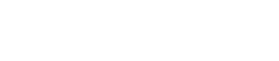October 14, 2023
October 14, 2023
October 14, 2023
###### GATE 2017 [Set-1]
October 14, 2023
 Question 1

Consider the following two statements about the function f(x)=|x|

```P. f(x) is continuous for all real values of x
Q. f(x) is differentiable for all real values of x ```

Which of the following is TRUE?

 A P is true and Q is false. B P is false and Q is true. C Both P and Q are true. D Both P and Q are false.
Question 1 Explanation:
f(x) = |x|
→ f(x) is continuous for all real values of xFor every value of x, there is corresponding value of f(x).
For x is positive, f(x) is also positive
x is negative, f(x) is positive.
So, f(x) is continuous for all real values of x.
→ f(x) is not differentiable for all real values of x.
For x<0, derivative is negative
x>0, derivative is positive.
Here, left derivative and right derivatives are not equal.

Question 1 Explanation:
f(x) = |x|
→ f(x) is continuous for all real values of xFor every value of x, there is corresponding value of f(x).
For x is positive, f(x) is also positive
x is negative, f(x) is positive.
So, f(x) is continuous for all real values of x.
→ f(x) is not differentiable for all real values of x.
For x<0, derivative is negative
x>0, derivative is positive.
Here, left derivative and right derivatives are not equal.